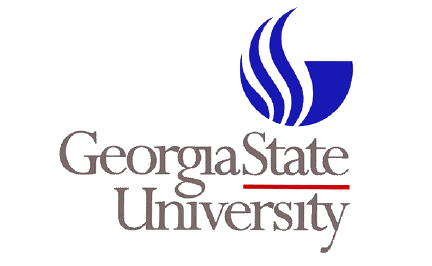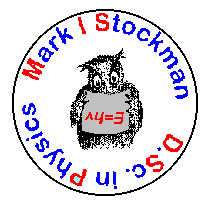Department of Physics and Astronomy

PHYS 6520/4520 (same as Math 6265/4265)
MATHEMATICS OF PHYSICS II
SUMMER SEMESTER 2001

Tuesday, Thursday 4:45 pm - 7:30 pm --- 527 General Classroom BuildingInstructor: Mark Stockman
Office: 455 Science Annex
Phone: (404)651-2779
E-mail: mstockman@gsu.edu
Internet: http://www.phy-astr.gsu.edu/stockman/

Grading: Homework 40%, midterm exam 20%, final exam 40%.
Final Exam: Friday, August 2 from 5 to 7 pm in the regular classroom (527 G)

Text: Erwin Kreysig, Advanced Engineering Mathematics (Eighth Edition), J. Wiley, New York, 1999

Prerequisite: Math 3260

SYLLABUS
1. Ordinary Differential Equations and  Special Functions (Chapter 4)
• Power series solutions
• Legendre equation and polynomials
• Frobenius method
• Bessel equation and functions
• Sturm-Liouville problem and orthogonal functions
• Solutions by orthogonal eigenfunction expansions
2. Fourier Series and Integrals (Chapter 10)
• Periodic functions
• Fourier series
• Even functions and Cosine series; Odd functions and Sine series
• Complex Fourier Series
• Fast Fourier transform (FFT)
• Example: Solving periodic and aperiodic mechanical problems using FFT
3. Partial Differential Equations (Chapter 11 with some material of Chapter 4)
• Introduction. Equations of mathematical physics: Hyperbolic (wave equation), parabolic (diffusion or heat equation and Schroedinger equation), and elliptic (Laplace equation and Poisson equation)
• Solving one-dimensional wave equation (vibrating spring): Separation of variables and Fourier expansion
• General solutions of differential equations: D'Alembert solution of the wave equation
• Diffusion equation: Solution by Fourier series and integrals.
• Two dimensional wave equation: Vibrating rectangular membrane
• Laplacian in polar coordinates. Use of Fourier-Bessel series. Vibrating circular membrane
• Laplace equation in three dimensions. Spherical Bessel functions, Legendre polynomials and  functions, and spherical harmonics. Potential problems
• Green's functions
4. Analytic Functions (Chapter 12) [Time permitting]
• Complex numbers, complex plane, modulus and argument
• Derivative, analytic functions
• Cauchy-Riemann and Laplace equations
• Conformal mapping
• Analytic properties of elementary functions: square root, exponential function, trigonometric and hyperbolic functions, and logarithm
• Branch cuts and Riemann surfaces
5. Complex integration (Chapter 13) [Time permitting]
• Complex line integral
• Cauchy theorem and integral
• Derivatives of analytic functions
•    6. Residue  integration (Chapter 15) [Time permitting]
• Laurent series
• Poles  and residues
• Residue integration method
• Evaluation of real integrals